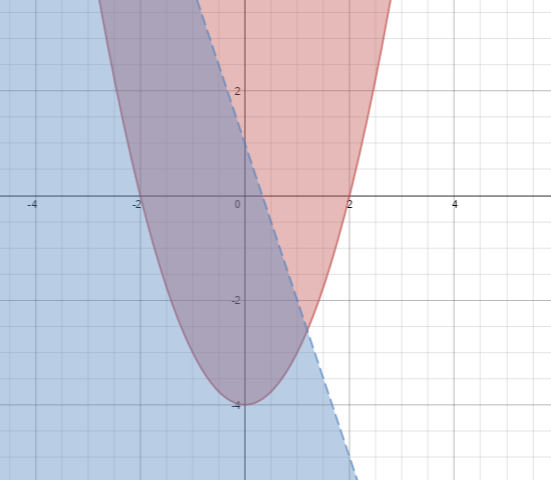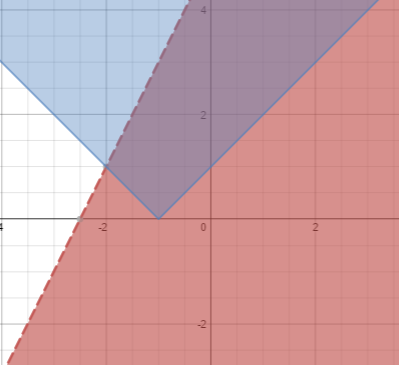### Home > CCA2 > Chapter 8 > Lesson 8.1.2 > Problem8-41

8-41.

Graph each system below and shade the solution region.

1. $y ≥ x^2 - 4$
$y < -3x + 1$

1. $y < 2x + 5$
$y ≥| x + 1 |$

Graph each function and decide if the lines should be dashed or solid. Then shade the appropriate region.Graph each function and decide if the lines should be dashed or solid. Then shade the appropriate region.